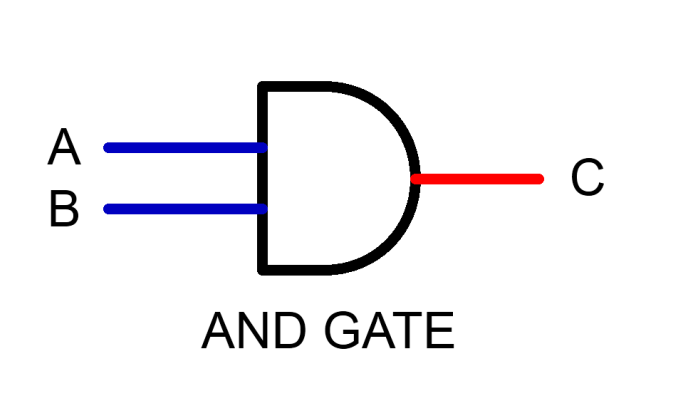# Follow These Easy Tips to Remember 4 Popular Logic Gates

0
689If you are studying physics, the electricity chapter will have a concept called the logic gates. Basically, logic gates are building blocks of a circuit that carries out logical operations. The functions on the Boolean algebra and most of them have two inputs and one output. The examples are AND Gate, NOR gate, and many others.

So, students who are studying the same in their higher studies often get issues about understanding its concepts. Therefore, we have brought this post highlighting a few ways to remember the logic gates operation.

# How to remember the logic gate?

• ## NOT gate

Let us start with NOT gate, which has a single input value. If you are putting the Boolean value true which is 1, then the output will be 0 (NOT True), which is the False Boolean value. Likewise, if you put the false value (0) then the output will be true (1). In simpler words, this gate will give the opposite output, whatever the input will be.

Solve a few questions on the same and practice a few circuit diagrams to get a good command of its concepts. Even solving curriculum books’ questions is enough to make your mastery in understanding this gate concept. Solve 10  or 15 questions and see that you will be a master in solving these logic gate questions.

• ## AND gate

Next, in this list is AND gate, where both values should be ‘True’ (1) to get the output ‘True’ in grammar language and means compounding two values to draw a conclusion. Therefore, if you want the ‘true’ output both the Boolean value should also be true. To make you understand in simpler words, here we present an example.

Jack is eating the burger, and Peter is having tea. Here both the items are mismatching, and therefore the output will not be true. Let us assume Jack (A) and Peter (B). If A=0 and B=1, then the output will be ‘0’, which means ‘False’. To make the output true, A =1 and B=1 should be there. In refreshers and curriculum textbooks, many questions are based on this logic gate. Solve as much as possible.

• ## XOR gate

The full form of XOR is ‘Exclusive OR.’ In simpler words, this logic gate will deliver the output ‘true’ if one Boolean value is false and the next one is true. Remember, if it contains both Boolean values the same, the result will be false.

• Examples: A=0 B=1 Result =1
• A=1 B=1 Result=0

In reality, we use the term ‘either’. Either you will crack an exam or you will do the job. It means you have to select one value only. So, this is what the fundamental is all about the XOR operations. Mostly, computers use this operating system to add binary codes in the system.

• ## NAND: The Complementary gate to know

The term NAND gate means the ‘NOT AND’ gate. This is a complementary gate that functions the same as the XOR gate. In this, if both values are ‘true, the output will be ‘False’. To understand it more in detail, we suggest you follow truth tables available online in textbooks. Also, there are several reference books available through which you can practice certain NAND and XOR gate questions.

## Final words

These gates are an important concept to learn before your board examination. To gain mastery over them, we suggest solving as many questions as possible. Also, remember about Boolean values and their operations while solving the logical operations. Attend online classes if there are any issues or else follow this post for more clarification.